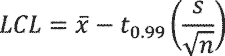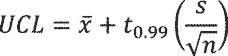# Title 10

Displaying title 10, up to date as of 9/21/2021. Title 10 was last amended 9/20/2021.

# Title 10

###### eCFR Content

The Code of Federal Regulations (CFR) is the official legal print publication containing the codification of the general and permanent rules published in the Federal Register by the departments and agencies of the Federal Government. The Electronic Code of Federal Regulations (eCFR) is a continuously updated online version of the CFR. It is not an official legal edition of the CFR.

§ 429.56 Integrated light-emitting diode lamps.

(a) Determination of Represented Value. Manufacturers must determine the represented value, which includes the certified rating, for each basic model of integrated light-emitting diode lamps by testing, in conjunction with the sampling provisions in this section.

(1) Units to be tested.

(i) The general requirements of § 429.11 (a) are applicable except that the sample must be comprised of production units; and

(ii) For each basic model of integrated light-emitting diode lamp, the minimum number of units tested must be no less than 10 and the same sample comprised of the same units must be used for testing all metrics. If more than 10 units are tested as part of the sample, the total number of units must be a multiple of two. For each basic model, a sample of sufficient size must be randomly selected and tested to ensure that:

(A) Represented values of initial lumen output, lamp efficacy, color rendering index (CRI), power factor, or other measure of energy consumption of a basic model for which consumers would favor higher values are less than or equal to the lower of:

(1) The mean of the sample, where:and, x is the sample mean; n is the number of units; and xi is the measured value for the ith unit; Or,

(2) The lower 99 percent confidence limit (LCL) of the true mean divided by 0.96; or the lower 99 percent confidence limit (LCL) of the true mean divided by 0.98 for CRI and power factor, where:and, x is the sample mean; s is the sample standard deviation; n is the number of samples; and t0.99 is the t statistic for a 99 percent one-tailed confidence interval with n-1 degrees of freedom (from appendix A to this subpart).

(B) Represented values of input power, standby mode power or other measure of energy consumption of a basic model for which consumers would favor lower values are greater than or equal to the higher of:

(1) The mean of the sample, where:and, x is the sample mean; n is the number of units; and xi is the measured value for the ith unit;

Or,

(2) The upper 99 percent confidence limit (UCL) of the true mean divided by 1.02, where:and, x is the sample mean; s is the sample standard deviation; n is the number of samples; and t0.99 is the t statistic for a 99 percent one-tailed confidence interval with n-1 degrees of freedom (from appendix A to this subpart);

(C) Represented values of correlated color temperature (CCT) of a basic model must be equal to the mean of the sample, where:and, x is the sample mean; n is the number of units in the sample; and xi is the measured CCT for the ith unit.

(D) The represented value of lifetime of an integrated light-emitting diode lamp must be equal to or less than the median time to failure of the sample (calculated as the arithmetic mean of the time to failure of the two middle sample units when the numbers are sorted in value order) rounded to the nearest hour.

(2) The represented value of life (in years) of an integrated light-emitting diode lamp must be calculated by dividing the lifetime of an integrated light-emitting diode lamp by the estimated annual operating hours as specified in 16 CFR 305.15(b)(3)(iii).

(3) The represented value of estimated annual energy cost for an integrated light-emitting diode lamp, expressed in dollars per year, must be the product of the input power in kilowatts, an electricity cost rate as specified in 16 CFR 305.15(b)(1)(ii), and an estimated average annual use as specified in 16 CFR 305.15(b)(1)(ii).

(b) Certification reports.

(1) The requirements of § 429.12 are applicable to integrated light-emitting diode lamps;

(2) Values reported in certification reports are represented values. Pursuant to § 429.12(b)(13), a certification report must include the following public product-specific information: The testing laboratory's NVLAP identification number or other NVLAP-approved accreditation identification, the date of manufacture, initial lumen output in lumens (lm), input power in watts (W), lamp efficacy in lumens per watt (lm/W), CCT in kelvin (K), power factor, lifetime in years (and whether value is estimated), and life (and whether value is estimated). For lamps with multiple modes of operation (such as variable CCT or CRI), the certification report must also list which mode was selected for testing and include detail such that another laboratory could operate the lamp in the same mode. Lifetime and life are estimated values until testing is complete. When reporting estimated values, the certification report must specifically describe the prediction method, which must be generally representative of the methods specified in appendix BB. Manufacturers are required to maintain records per § 429.71 of the development of all estimated values and any associated initial test data.

(c) Rounding requirements.

(1) Round input power to the nearest tenth of a watt.

(2) Round lumen output to three significant digits.

(3) Round lamp efficacy to the nearest tenth of a lumen per watt.

(4) Round correlated color temperature to the nearest 100 Kelvin.

(5) Round color rendering index to the nearest whole number.

(6) Round power factor to the nearest hundredths place.

(7) Round lifetime to the nearest whole hour.

(8) Round standby mode power to the nearest tenth of a watt.

[81 FR 43425, July 1, 2016]